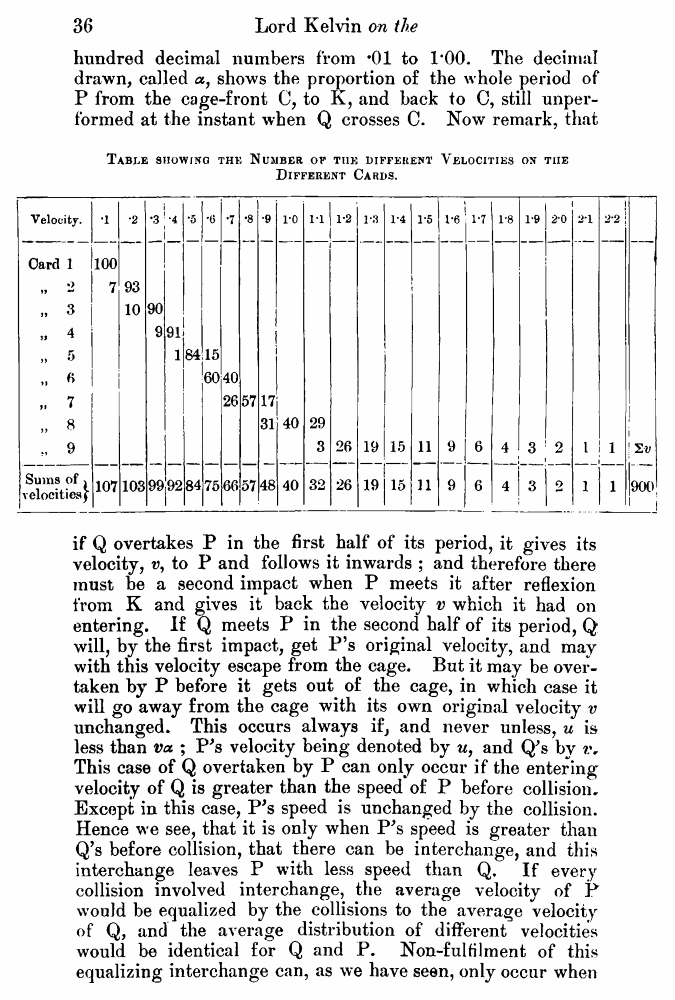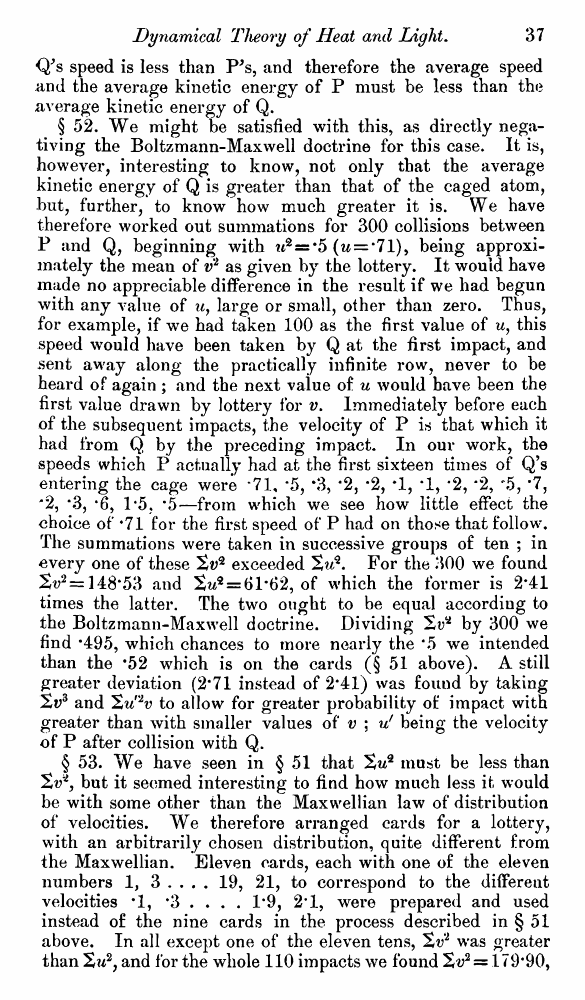﻿ ﻿ Lord Kelvin. Nineteenth-Century Clouds over the Dynamical Theory of Heat and Light. // Phil. Mag. S. 6. Vol. 2. No. 7. July 1901.

# Lord Kelvin. Nineteenth-Century Clouds over the Dynamical Theory of Heat and Light. // Phil. Mag. S. 6. Vol. 2. No. 7. July 1901.

В начало   Другие форматы   <<<     Страница 36   >>>1  2  3  4  5  6  7  8  9  10  11  12  13  14  15  16  17  18  19  20  21  22  23  24  25  26  27  28  29  30  31  32  33  34  35  36 37  38  39  40 hundred decimal numbers from *01 to 1*00. The decimal drawn, called a, shows the proportion of the whole period of P from the cage-front C, to K, and back to C, still unperformed at the instant when Q crosses C. Now remark, that Table showing the Number op the different Velocities on tiie Different Cards. if Q overtakes P in the first half of its period, it gives its velocity, v, to P and follows it inwards ; and therefore there must be a second impact when P meets it after reflexion from K and gives it back the velocity v which it had on entering. If Q meets P in the second half of its period, Q will, by the first impact, get P’s original velocity, and may with this velocity escape from the cage. But it may be overtaken by P before it gets out of the cage, in which case it will go away from the cage with its own original velocity v unchanged. This occurs always if, and never unless, u is less than va ; P's velocity being denoted by u, and Q's by This case of Q overtaken by P can only occur if the entering velocity of Q is greater than the speed of P before collision. Except in this case, P's speed is unchanged by the collision. Hence we see, that it is only when P's speed is greater than Q’s before collision, that there can be interchange, and this interchange leaves P with less speed than Q. If every collision involved interchange, the average velocity of P would be equalized by the collisions to the average velocity of Q, and the average distribution of different velocities would be identical for Q and P. Non-fulfilment of this equalizing interchange can, as we have seen, only occur when Q’s speed is less than P's, and therefore the average speed and the average kinetic energy of P must be less than the average kinetic energy of Q. § 52. We might be satisfied with this, as directly negativing the Boltzmann-Maxwell doctrine for this case. It is, however, interesting to know, not only that the average kinetic energy of Q is greater than that of the caged atom, but, further, to know how much greater it is. We have therefore worked out summations for 300 collisions between P and Q, beginning with w2=s*5 (w = *71), being approximately the mean of v2 as given by the lottery. It would have made no appreciable difference in the result if we had begun with any value of u, large or small, other than zero. Thus, for example, if we had taken 100 as the first value of u, this speed would have been taken by Q at the first impact, and sent away along the practically infinite row, never to be heard of again ; and the next value of u would have been the first value drawn by lottery for v. Immediately before each of the subsequent impacts, the velocity of P is that which it had from Q by the preceding impact. In our work, the speeds which P actually had at the first sixteen times of Q’s entering the cage were *71, *5, *3, *2, *2, *1, *1, *2, *2, “5, *7, '2, *3, *6, 1*5, *5—from which we see how little effect the choice of *71 for the first speed of P had on those that follow. The summations were taken in successive groups of ten ; in every one of these St;2 exceeded Xu*. For the 300 we found 2^*= 148*53 and 2w2 = 61#62, of which the former is 2*41 times the latter. The two ought to be equal according to the Boltzmann-Maxwell doctrine. Dividing by 300 we find *495, which chances to more nearly the *5 we intended than the *52 which is on the cards (§ 51 above). A still greater deviation (2*71 instead of 2*41) was found by taking 2v3 and 'Zu'2v to allow for greater probability of impact with greater than with smaller values of v ; v! being the velocity of P after collision with Q. § 53. We have seen in § 51 that %uq must be less than Sv*, but it seemed interesting to find how much less it would be with some other than the Maxwellian law of distribution of velocities. We therefore arranged cards for a lottery, with an arbitrarily chosen distribution, quite different from the Maxwellian. Eleven cards, each with one of the eleven numbers 1, 3 . . . . 19, 21, to correspond to the different velocities *1, *3 . . . . 1*9, 2*1, were prepared and used instead of the nine cards in the process described in § 51 above. In all except one of the eleven tens, was greater than %u2, and for the whole 110 impacts we found 2v2 = 179*90,